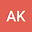On the completeness of metric spaces with a $$w$$-distance
•Abstract.  A $$w$$-distance on a metric space $$\left(X,d\right)$$ is a function $$p:X\times X\to\left[0,\infty\right)$$  which is lower semicontinuous with respect to the second varibale, satisfies the triangle inequality and for all $$\varepsilon>0$$ there exists a $$\delta>0$$ such that $$p\left(z,x\right)\le\delta$$ and $$p\left(z,y\right)\le\delta$$ imply $$d\left(x,y\right)\le\varepsilon$$ for all $$x,y,z\in X$$. In this short note we prove that a metric space with a $$w$$-distance $$p$$ is complete if and only if every sequence $$\ \left\{x_i\right\}\$$ such that $$\sum_{_{i=1}}^{\infty}p\left(x_i,x_{i+1}\right)<\infty$$ converges.